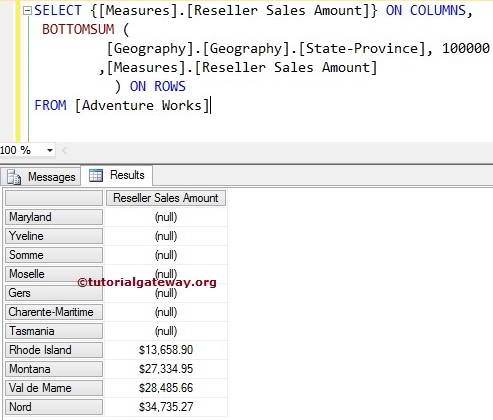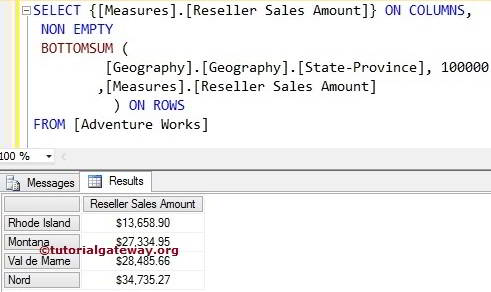# MDX BottomSum Function

The MDX BottomSum function will sort the given data in the Ascending order. And then, MDX bottomsum selects the required number of records from the sorted data whose total is at least equal to the specified value.

## MDX BottomSum Function Syntax

The basic syntax of the BottomSum in Multidimensional Expression is

`BOTTOMSUM (Set_Expression, Value, Numeric_Expression)`
• Set_Expression: Any Multidimensional Expression or attributes on which you want to check.
• Value: Please provide the Value. The MDX BOTTOMSUM function will retrieve the records whose total is at least this Value.
• Numeric_Expression: Any Multidimensional Expression or measure. The BottomSum function will use this Numeric_Expression to sort the data in ascending order. Next, it picks the number of records required to match the Value.

How to write MDX BottomSum function to extract the bottom States from Geography Dimension in the Adventure Works Cube whose total is at least equal to the user given value?. For this, we are going to use the below-shown data.

## MDX BottomSum Function Example

The following MDX bottomsum query will return Bottom States present in the Geography Dimension, who are contributing Sale of 100000 of total Reseller Sales Amount.

TIP: Please use TOPSUM Function to calculate the Sum of Top N records

```SELECT {[Measures].[Reseller Sales Amount]} ON COLUMNS,
BOTTOMSUM (
[Geography].[Geography].[State-Province]
,100000
,[Measures].[Reseller Sales Amount]
) ON ROWS

In the above MDX Query, We selected the [Reseller Sales Amount] Measure on the Columns and [State Province] column present in the geography Dimension on Rows. Next, the BottomSum function will sort the data in the Ascending order using Measures.[Reseller Sales Amount]. Then, add the Sales amount of each state until it reaches 100000.If you observe the above screenshot, If you total the Sales amount of above-displayed states, they approximately equal to 104214.78. It means greater than what we required.

## Using NON EMPTY in MDX BottomSum

In this example, we are going to use Non Empty keyword to remove the NULL values returned by the BottomSum function.

```SELECT {[Measures].[Reseller Sales Amount]} ON COLUMNS,
NON EMPTY
BOTTOMSUM (
[Geography].[Geography].[State-Province]
,100000
,[Measures].[Reseller Sales Amount]
) ON ROWSSuresh is the founder of TutorialGateway and a freelance software developer. He specialized in Designing and Developing Windows and Web applications. The experience he gained in Programming and BI integration, and reporting tools translates into this blog. You can find him on Facebook or Twitter.